Next: Propagation Matrix Up: C.1 One Homogeneous Planar Previous: Matrix Notation

### Matrix Factorization

For further investigations it is useful to split up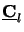(z) into two parts, namely into a constant matrix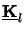describing the orientation of the wave propagation and into a z-dependent diagonal matrix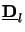(z) representing the amplitude oscillation within the layer. Hence we write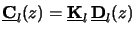(C.9)

with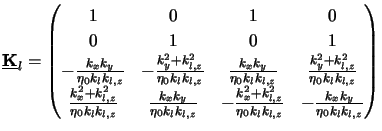(C.10)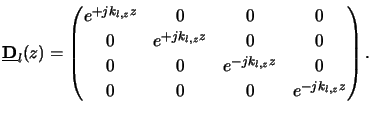(C.11)

Some interesting properties exist for the three matrices defined by (C.11) to (C.13),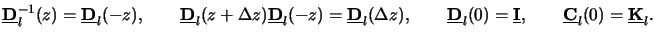(C.12)

The validity of each of these relations is self-evident. However, as they will be used further on we explicitly summarized them in (C.14). Additionally, the orientation matrixdefined in (C.12) can be factorized as follows: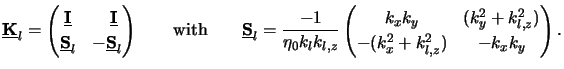(C.13)

Finally, the inverse matrix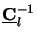(z) of(z) is of interest. From (C.11) and (C.13) we obtain with (C.14)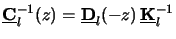(C.14)

whereby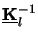is given by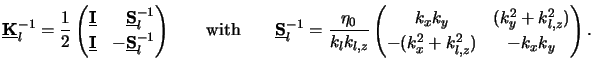(C.15)

Note thatsimply equals to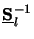= - 1/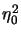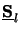.

Summarizing the results of the matrix notation and factorization (cf. (C.10), (C.11) and (C.16)) shows that the lateral field components ul(z) are related to the electric amplitudes el by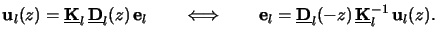(C.16)Next: Propagation Matrix Up: C.1 One Homogeneous Planar Previous: Matrix Notation
Heinrich Kirchauer, Institute for Microelectronics, TU Vienna
1998-04-17